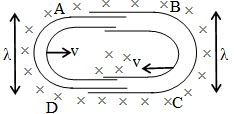# Topic Electromagnetic Induction, Class 12 Physics MCQ Quiz Test

#### How to Count Your Score

You will get 1 point for each correct answer. At the end of the Quiz, your total score will be displayed. Maximum score is 30 points.

Good Luck, Lets start the Quiz on Physics Topic Electromagnetic Induction

MCQ 1:
The instantaneous flux associated with a closed circuit of 10Ω resistance is indicated by the following reaction φ = 6t2 – 5t + 1, then the value in amperes of the induced current at t = 0.25 sec will be -

MCQ 2:
A cylindrical bar magnetic is lying along the axis of a circular coil. If the magnet is rotated about the axis of the coil then-

MCQ 3:
When a coil of area 2 cm2 and having 30 turns, whose plane is normal to the magnetic field, is drawn out of the magnetic field, a charge of 1.5 × 10–4 coulomb flows in the circuit. If its resistance is 40 ohm, then the magnetic flux density in Tesla will be –

MCQ 4:
When a magnet is being moved towards a coil, the induced emf does not depend upon -

MCQ 5:
A wire carrying current I, lie on the axis of a conducting ring. The direction of the induced current in the ring, when I is decreasing at a steady rate is -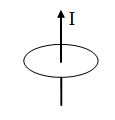MCQ 6:
A flux of 1m Wb passes through a strip having an area A = 0.02 m2. The plane of the strip is at an angle of 60° to the direction of a uniform field B. The value of B is-

MCQ 7:
A small loop of area of cross section 10–4 m2 is lying concentrically and coplanar inside a bigger loop of radius 0.628m. A current of 10A is passed in the bigger loop. The smaller loop is rotated about is diameter with an angular velocity ω. The magnetic flux linked with the smaller loop will be -

MCQ 8:
A coil of N turns and area A is rotated at the rate of n rotations per second in a magnetic field of intensity B, the magnitude of the maximum magnetic flux will be -

MCQ 9:
Lenz's law is consistent with law of conservation of -

MCQ 10:
The north pole of a magnet is brought near a coil. The induced current in the coil as seen by an observer on the side of magnet will be-

MCQ 11:
A magnetic field is directed normally downwards through a metallic frame as shown in the figure. On increasing the magnetic field -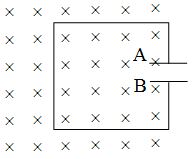MCQ 12:
Two coils P and Q are lying a little distance apart coaxially. If a current I is suddenly set up in the coil P then the direction of current induced in coil Q will be -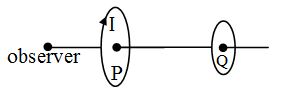MCQ 13:
A system S consists of two coils A and B. The coil A carries a steady current I while the coil B is suspended near by as shown in fig. Now if the system is heated so as to raise the temperature of two coils steadily then -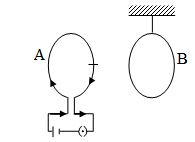MCQ 14:
Consider the situation shown in fig. If the current I in the long straight wire XY is increased at a steady rate then the induced emf's in loops A and B will be -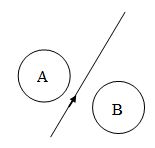MCQ 15:
A rectangular loop of resistance R, and sides I and x, is pulled out of a uniform magnetic field B with a steady velocity v. The necessary force F required for maintaining uniform velocity of withdrawal is -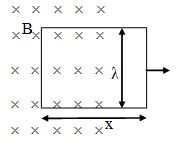MCQ 16:
A small conducting rod of length λ, moves with a uniform velocity v in a uniform magnetic field B as shown in figure given below -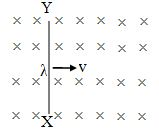MCQ 17:
A conductor ABOCD moves along its bisector with a velocity of 1 m/s through a perpendicular magnetic field of 1 Wb/m2, as shown in fig. If all the four sides are of 1m length each, then the induced emf between points A and D is -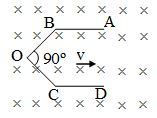MCQ 18:
A circular coil of radius r is placed in a uniform magnetic field B. The magnetic field is normal to the plane of the coil, as shown in fig. Now if the coil is rotated at an angular speed of ω, about its own axis, then the induced emf in the coil is -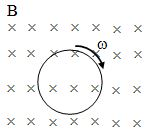MCQ 19:
An electric potential difference will be induced between the ends of the conductor shows in fig, when conductor moves in the direction -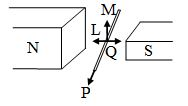MCQ 20:
The significance of self inductance L is the same as of that of ___________ in the linear motion -

MCQ 21:
On making a circular coil of copper wire of length λ and coil radius r, the value of self inductance is obtained as L. If the coil of same wire, but of coil radius r/2, is made, the value of self inductance will be-

MCQ 22:
Out of the two coils placed near each other, when a current of 2 amp is passed in one, a flux of 6 × 10–5 Weber passes through the other. If the number of turns in the secondary coils is 20, the value of coefficient of mutual induction in the coils will be -

MCQ 23:
The coefficient of mutual induction between two coils is 4H. If the current in the primary reduces from 5A to zero in 10–3 second then the induced e.m.f. in the secondary coil will be -

MCQ 24:
The coefficient of mutual induction between two closely lying coils does not depend upon -

MCQ 25:
The number of turns in a coil of wire of fixed radius is 600 and its self inductance is 108 mH. The self inductance of a coil of 500 turns will be -

MCQ 26:
The value of the self inductance of a coil is 5 H. If the current in the coil changes steadily from 1A to 2A in 0.5 seconds, then the magnitude of induced emf is-

MCQ 27:
An inductance stores energy in -

MCQ 28:
A thin copper wire of length 100 metres is wound as a solenoid of length λ and radius r. Its self inductance is found to be L. Now if the same length of wire is wound as a solenoid of length λ but of radius r/2, then its self inductance will be -

MCQ 29:
When a rectangular loop pulled out from uniform magnetic field. The induced current is proportional to -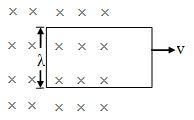MCQ 30:
One conducting U tube can slide inside another as shown in figure, maintaining electrical contacts between the tubes. The magnetic field B is perpendicular to the plane of the figure. If each tube moves towards the other at a constant speed V, then the emf induced in the circuit in terms of B, λ and V where λ is the width of each tube, will be –